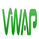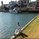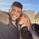127952 views
Supertrend Indicator with Buy and Sell Indicators. Supertrend is quite a popular indicator. To know more about it check out the article below.

10 Things to know about Supertrend
http://www.marketcalls.in/amibroker/10-t...
```//Author - Rajandran R
//www.marketcalls.in
study("Supertrend V1.0 - Buy or Sell Signal", overlay = true)

Factor=input(3, minval=1,maxval = 100)
Pd=input(7, minval=1,maxval = 100)

Up=hl2-(Factor*atr(Pd))
Dn=hl2+(Factor*atr(Pd))

TrendUp=close>TrendUp? max(Up,TrendUp) : Up
TrendDown=close<TrendDown? min(Dn,TrendDown) : Dn

Trend = close > TrendDown ? 1: close< TrendUp? -1: nz(Trend,1)
Tsl = Trend==1? TrendUp: TrendDown

linecolor = Trend == 1 ? green : red

plot(Tsl, color = linecolor , style = line , linewidth = 2,title = "SuperTrend")

plotshape(cross(close,Tsl) and close>Tsl , "Up Arrow", shape.triangleup,location.belowbar,green,0,0)
plotshape(cross(Tsl,close) and close<Tsl , "Down Arrow", shape.triangledown , location.abovebar, red,0,0)
//plot(Trend==1 and Trend==-1,color = linecolor, style = circles, linewidth = 3,title="Trend")

plotarrow(Trend == 1 and Trend == -1 ? Trend : na, title="Up Entry Arrow", colorup=lime, maxheight=60, minheight=50, transp=0)
plotarrow(Trend == -1 and Trend == 1 ? Trend : na, title="Down Entry Arrow", colordown=red, maxheight=60, minheight=50, transp=0)

```Hi Rajandran,
I love this indicator. Is there a way to add an offset function? it seems the indicator correctly indicates market changes but is 6 bars late. An offset function could allow a more precise read on the market trend.